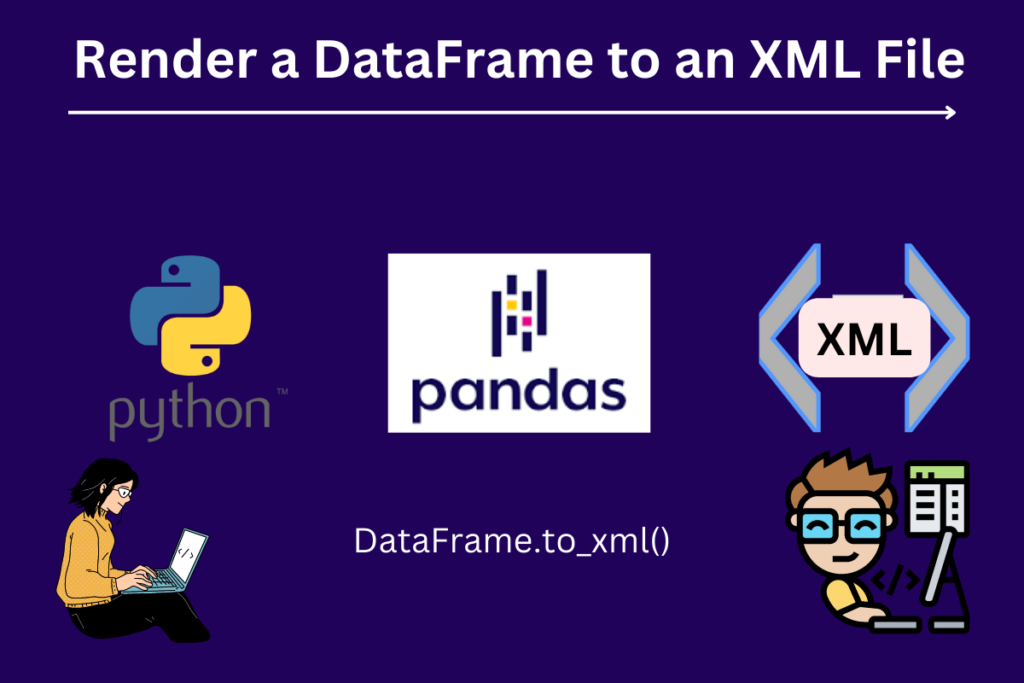# Pandas DataFrame.to_xml – Render a DataFrame to an XML DocumentPandas is a fast, robust, flexible, easy-to-use open-source data analysis and manipulation tool built on Python. It is a Python library that is used for analyzing data.

`pandas.DataFrame()` is a 2-dimensional data structure just like an array or a table with rows and columns.

Here we are learning how to turn the pandas DataFrame into an XML format or document. To do so, we have the method DataFrame.to_xml(). XML stands for eXtensible Markup Language; it is similar to HTML. XML does not have predefined tags; instead, one can design specific tags as per the need. `DataFrame.to_xml() `method encodes the tabular data into a readable format.

You may check: Working with DataFrame Rows and Columns in Python

## Syntax of to_xml()

```DataFrame.to_xml(path_or_buffer=None, index=True, root_name='data', row_name='row', na_rep=None, attr_cols=None, elem_cols=None, namespaces=None, prefix=None, encoding='utf-8', xml_declaration=True, pretty_print=True, parser='lxml', stylesheet=None, compression='infer', storage_options=None)
```

### Parameter List

Some parameters are:

• path_or_buffer: string, path object (implementing `os.PathLike[str]`), or file-like object implementing a `write()` function. If None, the result is returned as a string.
• index: whether to include an index in XML document(bool)
• row_name: the name of the row element in XML document.
• attr_cols: list of columns to write as attributes in a row element.
• namespaces: all namespaces are to be defined in the root element. Keys of the dictionary should be prefix names and values of dictionary corresponding URIs

## Install Pandas and lxml

Before getting into the coding part, install the Pandas library, which will provide us with its methods and attributes like `read_csv()`, `loc[:]`, `to_datetime()`, `merge()`, `to_csv()`, `to_xml()`, and so on.

```pip install pandas
```

Install lxml provides safe and convenient access to these libraries using the ElementTree API. It extends the ElementTree API significantly to support XPath, RelaxNG, XML Schema, XSLT, C14N, and more.

```pip install lxml
```

## Render an XML Document

### Example 1: Basic Dataframe from dictionary to XML file.

```import pandas as pd
```

After importing the Pandas module, let’s write the simple code to render the DataFrame to an XML file. DataFrame takes in the dictionary with the key-value pair.

```import pandas as pd

df = pd.DataFrame({
'Name': ['Dhushi', 'Praj', 'John', 'Lee'],
'Age' : [6, 26, 50, 32]
})

df.to_xml('file.xml')
```
1. import the Pandas library as pd, which will give access to the methods and functions
2. save the DataFrame object in the variable ‘df
3. `to_xml()` method to turn the dataframe into the XML file
4. ‘file.xml’ is a filename where output is stored.

Note: use .xml file extension

#### Output:

In the output file, which is file.xml, we got the tags like data, row, index, name, and age created with the methods as we can see above.

### Example 2: to_xml() with attr_cols parameter

attr_cols parameter takes a list of columns to write as attributes in a row element. Hierarchical columns will be flattened with an underscore delimiting the different levels.

```import pandas as pd

df = pd.DataFrame({
'Name' : ['Dhushi', 'Praj', 'John', 'Lee'],
'Age' : [6, 26, 50, 32]
})

df.to_xml('data.xml', attr_cols=['index', 'Name', 'Age'])
```
• save the DataFrame object in the variable ‘df
• `to_xml()` method to turn the dataframe into the XML file
• ‘data.xml’ is a filename where output is stored
• provided the parameter attr_cols, which takes the list of columns- index, Name, and Age

#### Output:

As we can see in the output, the columns index, name, and age become an attribute of the row. The row becomes the self-closing tag.

### Example 3: to_xml() with namespaces parameter

namespaces parameter can be defined in the root element. Keys of the dictionary should be prefix names and values of dictionary corresponding URIs. Default namespaces should be given an empty string key.

```import pandas as pd

df = pd.DataFrame({
'Name' : ['Dhushi', 'Praj', 'John', 'Lee'],
'Age' : [6, 26, 50, 32]
})

df.to_xml('data.xml',
namespaces={"doc": "https://example.com"},
prefix="doc"
)
```
• save the DataFrame object in the variable ‘df
• `to_xml()` method to turn the dataframe into the XML file
• ‘data.xml’ is a filename where output is stored
• provided the parameter namespaces, which take a dictionary where the URL is in the value, and the key is the prefix

#### Output:

The advantage of using `DataFrame.to_xml`() can encode complex tabular data in a readable format, making it simple to share data with other applications and easily encode nested data structures, so it is ideal for representing data with multiple hierarchical levels. Its main drawback is that XML documents tend to be bulky and can be slow to process because of their complexity.
This article taught us how to convert a DataFrame to an XML document, examples containing parameters, and what they offer us. The `attr_cols` parameter makes a list of columns to be written as attributes in a row element, and with the `namespaces` parameter, we can add the URI as a value of the dictionary. More parameters can be used for different functionalities and purposes accordingly.